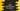# How to create a hollow diamond star pattern in C## How to create a hollow diamond star pattern in C:

In this post, we will learn how to write a program in C to print a hollow diamond star pattern. The program will take the height of the pattern as input from the user and print the pattern.

I will show you how to create the hollow star pattern using star or any other character and also I will explain to you the algorithm to draw it. If you understand the algorithm, you can easily write down it in code.

### What we are printing:

We will print a hollow diamond pattern. For example, the below pattern is a hollow diamond pattern of height 6:

``````     *
* *
*   *
*     *
*       *
*         *
*       *
*     *
*   *
* *
*``````

We can break it into two triangles. The upper triangle:

``````     *
* *
*   *
*     *
*       *
*         *``````

and the lower triangle:

`````` *       *
*     *
*   *
* *
*``````

So, the program will print the upper triangle and then it will print the lower triangle.

### Printing the upper triangle:

Both triangles are printed in four steps:

• Print spaces
• Print *
• Print spaces
• Print *

We need to decide how many spaces we need to print before and in between the stars.

Let’s take a look at the below triangle for total row 5:

``````    *
* *
*   *
*     *
*       *``````

If I use # to replace the spaces before * and & to replace the spaces in between *, it will look as like below:

``````####*
###*&*
##*&&&*
#*&&&&&*
*&&&&&&&*``````

This can be print by using the below code snippet:

``````for (i = 0; i < r; i++)
{
for (j = r - 1; j > i; j--)
{
printf("#");
}
printf("*");
for (j = 0; j <= (i - 1) * 2; j++)
{
printf("&");
}
if (i == 0)
{
printf("\n");
}
else
{
printf("*\n");
}
}``````

Here, r is the number of rows.

### Printing the lower triangle:

Let’s use the same approach to print the lower triangle:

``````#*&&&&&*
##*&&&*
###*&*
####*``````

It is also for total row 5.

We can use the below code to print this triangle:

``````for (i = r - 1; i > 0; i--)
{
for (j = r; j > i; j--)
{
printf("#");
}
printf("*");
for (j = 1; j < (i - 1) * 2; j++)
{
printf("&");
}
if (i == 1)
{
printf("\n");
}
else
{
printf("*\n");
}
}``````

Where, r is the total number of rows.

### Complete program:

We can combine both of these programs to print the whole triangle. The below program takes the row value as input from the user and prints the complete hollow diamond star pattern:

``````#include <stdio.h>
#include <stdlib.h>

int main()
{
int i, j, r;
printf("Enter the value of rows: ");
scanf("%d", &r);

for (i = 0; i < r; i++)
{
for (j = r - 1; j > i; j--)
{
printf(" ");
}
printf("*");
for (j = 0; j <= (i - 1) * 2; j++)
{
printf(" ");
}
if (i == 0)
{
printf("\n");
}
else
{
printf("*\n");
}
}

for (i = r - 1; i > 0; i--)
{
for (j = r; j > i; j--)
{
printf(" ");
}
printf("*");
for (j = 1; j < (i - 1) * 2; j++)
{
printf(" ");
}
if (i == 1)
{
printf("\n");
}
else
{
printf("*\n");
}
}

return 0;
}``````

If you run this program, it will print output as like below:

``````Enter the value of rows: 6
*
* *
*   *
*     *
*       *
*         *
*       *
*     *
*   *
* *
*

Enter the value of rows: 5
*
* *
*   *
*     *
*       *
*     *
*   *
* *
*``````

### Write the program using while loop:

We can also write it using while loop. While loop works similar to for loops. The only difference is that we need to write the initial condition and end condition before the loop starts and after each iteration.

The below program uses only while loops to print diamond star patterns:

``````#include <stdio.h>
#include <stdlib.h>

int main()
{
int i = 0, j, r;
printf("Enter the value of rows: ");
scanf("%d", &r);

while (i < r)
{
j = r - 1;
while (j > i)
{
printf(" ");
j--;
}

printf("*");

j = 0;
while (j <= (i - 1) * 2)
{
printf(" ");
j++;
}

if (i == 0)
{
printf("\n");
}
else
{
printf("*\n");
}

i++;
}

i = r - 1;
while (i > 0)
{
j = r;
while (j > i)
{
printf(" ");
j--;
}

printf("*");

j = 1;
while (j < (i - 1) * 2)
{
printf(" ");
j++;
}

if (i == 1)
{
printf("\n");
}
else
{
printf("*\n");
}
i--;
}

return 0;
}``````

You will get similar output if you run this program:

``````Enter the value of rows: 10
*
* *
*   *
*     *
*       *
*         *
*           *
*             *
*               *
*                 *
*               *
*             *
*           *
*         *
*       *
*     *
*   *
* *
*``````